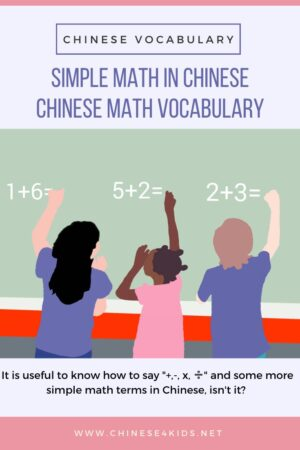# Simple Math in Chinese: Learn some math vocabulary in ChineseWe use numbers nearly everyday. Actually we use simple math everyday. Do you know how to say math in Chinese? How about we learn some math vocabulary together?

Math in Chinese is 数学(shùxué).

## 基本计算词汇 jīběn jìsuàn cíhuì

–   减 jiǎn subtract, minus, subtraction
✕ 乘 chéng times, multiply, multiplication
➗ 除 chú divide, division
= 等于 děngyú equal to

Let’s look at some examples:

3+7=10 三加七等于十 Sān jiā qī děngyú shí
7-4=3 七减四等于三 Qī jiǎn sì děngyú sān
3✕7=21 三乘以七等于二十一 Sān chéng yǐ qī děngyú èrshíyī
21➗7=3 二十一除以七等于三 Èrshíyī chú yǐ qī děngyú sān

## Must-know Math Words and Phrases in Chinese

If you don’t know the basic Chinese numbers, read article ” Why Is Learning Chinese Numbers Important” or grab the Chinese Number Booklet to find out how easy and useful numbers are in Chinese!

Zhōngwén shùzì hěn jiǎndān.
Numbers in Chinese are quite simple.

### 奇数 and 偶数 odd and even numbers

Shùzì 3, 5, 7, 9 dōu shì jīshù.
Numbers 3, 5, 7, 9 are all odd numbers.

Shùzì 4, 6, 8, 10 dōu shì ǒushù.
Numbers 4, 6, 8 and 10 are all even numbers.

### 正数 and 负数 positive and negative numbers

100是个正数。
100 Shìgè zhèng shù.
100 is a positive number.

-100是个负数。
-100 Shìgè fùshù.
-100 is a negative number.

### 整数, 分数, 小数 and 百分比 whole number, fraction, decimal, and percent

11是个整数。
11 Shìgè zhěngshù.
11 is a whole number.

Sì fēn zhī yī Shìgè fēnshù.
¼ is a fraction.

1.2是个小数。
Yī diǎn èr shìgè xiǎoshù.
1.2 is a decimal.

Yībǎi de bǎi fēn zhī shí shì shí.
10% of 100 is 10.

Let’s have a better look at how to say fractions, decimals and percents.

For a fraction, a/b, a is 分子 (Numerator) in Chinese and b is 分母(Denominator). In Chinese, we say a/b as b 分之a.

So, for the example of ¼, we can say “四分之一”.

⅗ is “五分之三” Wǔ fēn zhī sān.

½ is a bit special, we can either say “二分之一” （èr fēn zhī yī）or “一半”（yī bàn）.

For a decimal, the point is called “小数点”. Let’s look at several decimals.

0.2 零点二 líng diǎn èr
1.78 一点七八 yī diǎn qībā
13.02 十三点零二 shísān diǎn líng èr
204.35 二百零四点三五 èr bǎi líng sì diǎn sānwǔ

For a percentage, we just need to put “百分之” （bǎi fēn zhī）in front of the number, such as,

12% 百分之十二 bǎi fēn zhī shí’èr
50% 百分之五十 bǎi fēn zhī wǔshí
100% 百分之一百 bǎi fēn zhī yībǎi

### 大于 and 小于

100>99 一百大于九十九 yībǎi dàyú jiǔshíjiǔ
98<99 九十八小于九十九 jiǔ shí bā xiǎoyú jiǔ shí jiǔ

Look, we pretty much talked about all the key vocabulary of simple math in Chinese. Isn’t it pretty easy?

If you like this post, PIN IT!Resources mentioned:

Share it